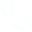# Python: Five Cases to Learn Class Attributes

Case explanation
1. Design two classes:
A point class, attributes include x, y coordinates.
A Rectangle class (rectangle), the property has the coordinates of the upper left corner and the lower right corner
Method: 1. Calculate the area of the rectangle; 2. Determine whether the point is within the rectangle
Instantiate a point object, a square object, output the area of the rectangle, and the output point is within the rectangle

class Point(object):
# When the Point method is created, it needs two parameters of type int to represent the x and y coordinates
def __init__(self, x: int, y: int):
self.x = x
self.y = y

class Rectangle(object):
def __init__(self, top_left: Point, bottom_right: Point):
self. top_left = top_left
self.bottom_right = bottom_right

def get_area(self):
# area: length * width
length = abs(self. bottom_right. x - self. top_left. x)
width = abs(self.top_left.y - self.bottom_right.y)
return length * width

def is_inside(self, point):
# if self.bottom_right.x >= point.x >= self.top_left.x and self.top_left.y >= point.y >= self.bottom_right.y:
# return True
# else:
# return False
return self.bottom_right.x >= point.x >= self.top_left.x and self.top_left.y >= point.y >= self.bottom_right.y

p1 = Point(4, 20) # define the point of the upper left corner
p2 = Point(30, 8) # define the point of the lower right corner

r = Rectangle(p1, p2) # pass the top left and bottom right points to the rectangle
print(r. get_area()) # 312

# p = Point(10;, 13) # True
p = Point(20;, 30) # False
print(r.is_inside(p))
2. Write a calculator class that can perform addition, subtraction, multiplication, and division calculations

class Calculator(object):
@staticmethod
return a + b

@staticmethod
def sub(a, b):
return a - b

@staticmethod
def mul(a, b):
return a * b

@staticmethod
def div(a, b):
return a / b

print(Calculator. sub(4, 5)) # -1
print(Calculator.mul(4, 5)) # 20
print(Calculator. div(4, 5)) # 0.8
3. Create a Person class and add a class field to count the number of objects of the Person class

class Person(object):
__count = 0 # class attributes

def __new__(cls, *args, **kwargs):
x = object.__new__(cls) # Apply for memory, create an object, and set the type to be Person class
return x

def __init__(self, name, age):
Person.__count += 1
self.name = name
self. age = age

@classmethod
def get_count(cls):
return cls.__count

# Every time an object is created, the __new__ and __init__ methods are called
# Call the __new__ method to apply for memory
# If you don't override the __new__ method, it will automatically find the object's __new__
# The __new__ method of object, the default implementation is to apply for a piece of memory and create an object
p1 = Person('Zhang San', 18)
p2 = Person('Li Si', 19)
p3 = Person('jack', 20)

# print(Person. count) # 3

print(p1, p2, p3)
print(Person. get_count()) # 3

# p4 = object.__new__(Person) # Apply for memory, create an object, and set its type to Person
# p4.__init__('tony', 23)
# print(p4)
4. Create a car class Auto, including the number of tires, car color, body weight, speed and other attributes, and create instances through different construction methods. At least the car is required to be able to accelerate, decelerate, and stop. Then define a car class CarAuto to inherit Auto and add air conditioning and navigation attributes, and reimplement methods to override acceleration and deceleration methods

class Auto(object):
def __init__(self, color, weight, speed=0, wheel_count=4):
self.wheel_count = wheel_count
self.color = color
self.weight = weight
self.speed = speed

def change_speed(self, x):
"""
modify speed
:param x: Indicates the vehicle speed value to be modified. If it is a positive number, it means acceleration, a negative number means deceleration, and 0 means stop
"""
if x == 0:
self.speed = 0 # If the passed parameter is 0, it means to stop
return
self.speed += x
if self.speed <= 0 and x < 0
return # No data can be added after return, indicating the end of the function

class CarAuto(Auto):
def __init__(self, color, weight, ac, navigator, speed=0, wheel_count=4):
super(CarAuto, self).__init__(color, weight, speed, wheel_count)
self.navigator = navigator
self.ac = ac

car = CarAuto('White', 1.6, 'Midea', 'Android', 10, 5)

car. change_speed(30)
print(car. speed) # 30

car. change_speed(-40)
print(car.speed) # 0
5. There is a bank account class Account, including attributes such as name and balance, and the methods include operations of depositing money, withdrawing money, and querying balance. Require:
1. When depositing money, pay attention to the format of the deposit data
2. When withdrawing money, it is necessary to judge whether the balance is sufficient, and when the balance is not enough, it is necessary to remind that the balance is insufficient

class Account(object):
def __init__(self, name, balance):
self.name = name
self. balance = balance

def save_money(self, money):
assert isinstance(money, float) or isinstance(money, int), 'Please pay attention to the format of the deposit data'
self.balance += money

def take_money(self, money):
if self. balance < money:
print('Insufficient balance')
else:
self. balance -= money

def check_balance(self):
return self. balance

a = Account('Zhang San', 8000)
a. save_money(10000)
a. take_money(1000)
print('current balance:', a.check_balance())
a. take_money(20000)
print('current balance:', a.check_balance())

## Related Articles

• ### A detailed explanation of Hadoop core architecture HDFS

Knowledge Base Team

• ### What Does IOT Mean

Knowledge Base Team

• ### 6 Optional Technologies for Data Storage

Knowledge Base Team

• ### What Is Blockchain Technology

Knowledge Base Team

## Explore More Special Offers

1. ### Short Message Service(SMS) & Mail Service

50,000 email package starts as low as USD 1.99, 120 short messages start at only USD 1.00Contact Us